# CIE A Level Maths: Probability & Statistics 1复习笔记1.1.4 Coding

### Coding

Sometimes data needs to be coded for further use with calculations. This is particularly useful with data that deals with very small or very large numbers, or with data that needs to be classified for research purposes.

#### What is coding?

• Coding is a way of simplifying data to make it easier to work with
• The coding must be carried out on all values within the data set and will normally be done using a given formula
• Coding can be carried out in a number of ways:
• Adding or subtracting a constant to each data value
• Multiplying or dividing each data value by a constant
• A combination of both of the above
• You may occasionally see coding called an assumed mean
• This refers to the value chosen to subtract from the original data
• It is usually chosen as an easy value, similar to an estimate of the true mean

#### How are statistical calculations carried out with coded data?

• If you know the mean or standard deviation of the original data it is possible to find the mean or standard deviation of the coded data and vice versa
• It is important to remember what the mean and standard deviation actually tell us about the data to understand how coding calculations work
• The mean is a measure of location, changing the data set in any way will cause the mean to change in the same way
• The standard deviation is a measure of spread, adding or subtracting a constant to every value within the data set will not change the standard deviation of the data set
• Multiplying or dividing every value within the data set by a constant will change the standard deviation by the modulus of the constant
• If the data were coded by multiplying or dividing by a negative, the standard deviation will change by the equivalent positive value
• Anytime calculations are carried out on data that has been coded,
• The original mean can be found by solving the equation to reverse the coding

####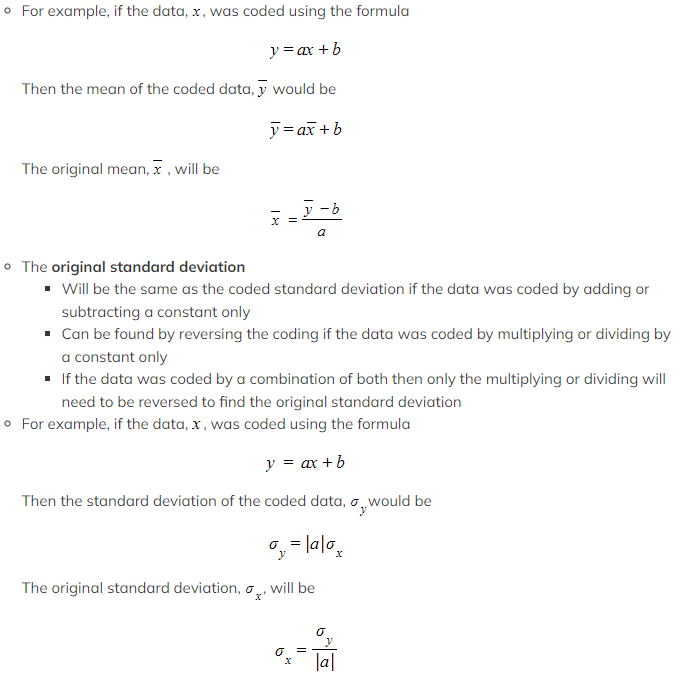What will summary statistics and formulae look like with an assumed mean?

• If an assumed meana, has been subtracted from all data values, x, then the summary statistics for the coded data will be
•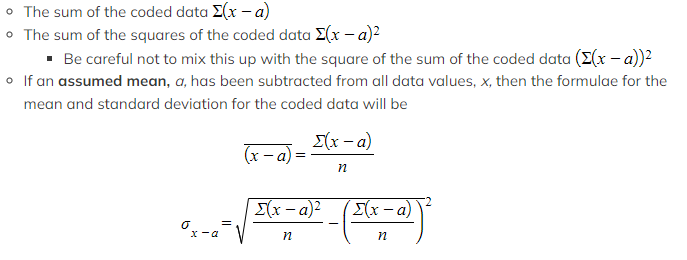Most questions will give you either the summary statistics or the mean of the coded data and expect you to work with these formulae to find original information about the data

#### Worked Example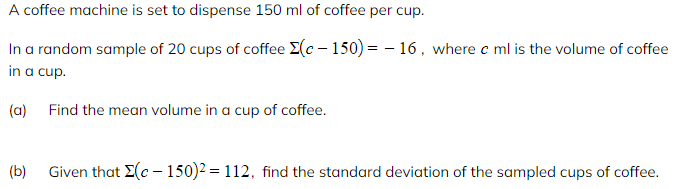(a)Find the mean volume in a cup of coffee.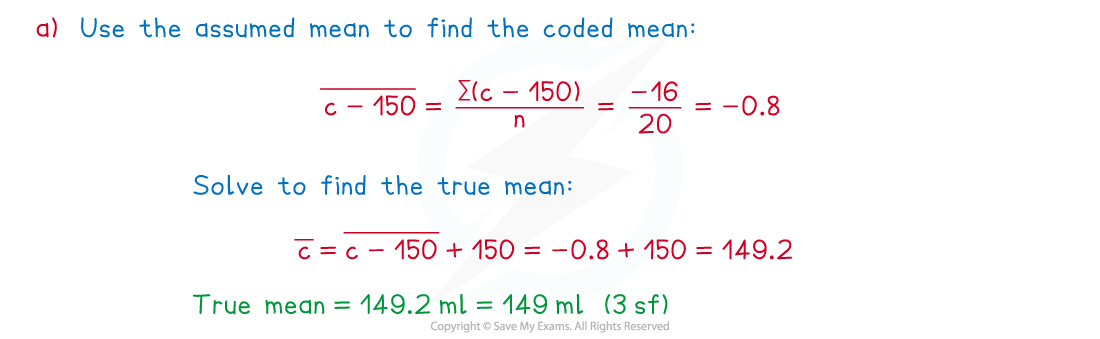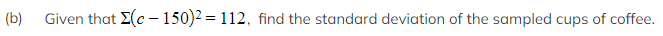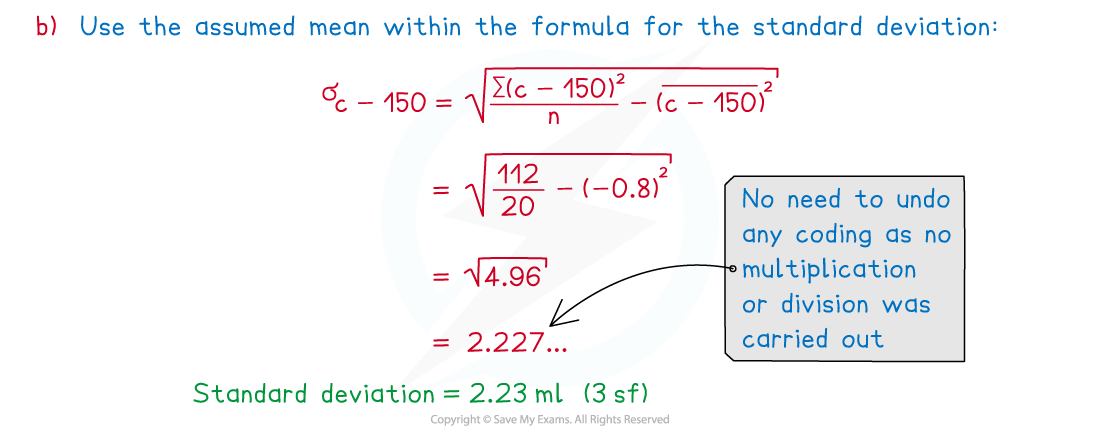#### Exam Tip

• Be careful when using the formulae for the mean and standard deviation with coded summary statistics, you must make sure that you use the summary statistics consistently throughout. For example, if you use the sum of the coded data squared in the formula for the standard deviation, you must subtract the square of the coded mean.